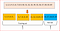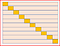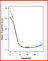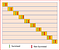# K fold and other cross-validation techniques

Here we will understand what is cross-validation and why we use it, different variations of cross-validation including K fold cross-validation.

Prerequisites: Machine Learning basics, understanding bias and variance, and how to evaluate a model’s performance

In Machine learning, we usually divide the dataset into Training dataset, Validation dataset, and Test dataset.

Training data set — used to train the model, it can vary but typically we use 60% of the available data for training.

Validation data set — Once we select the model that performs well on training data, we run the model on validation data set. This is a subset of the data usually ranges from 10% to 20%. Validation data set helps provide an unbiased evaluation of the model’s fitness. If the error on the validation dataset increases then we have an overfitting model.

Test dataset — Also called as holdout data set. This dataset contains data that has never been used in the training. Test data set helps with final model evaluation. Typically would be 5% to 20% of the dataset.

Sometimes there can be only training and test set and no validation set.

What’s the problem with this approach?

• Due to sample variability between training and test set, our model gives a better prediction on training data but fail to generalize on test data. This leads to a low training error rate but a high test error rate.
• When we split the dataset into training, validation and test set, we use only a subset of data and we know when we train on fewer observations the model will not perform well and overestimate the test error rate for the model to fit on the entire dataset

## To solve the two issue we use an approach called cross-validation

Cross-validation is a statistical technique which involves partitioning the data into subsets, training the data on a subset and use the other subset to evaluate the model’s performance.

To reduce variability we perform multiple rounds of cross-validation with different subsets from the same data. We combine the validation results from these multiple rounds to come up with an estimate of the model’s predictive performance.

Cross-validation will give us a more accurate estimate of a model’s performanceCross Validation — partition 20 data points into 4 subsets, train on 3 subsets and test on 1 subset

Let’s now understand few common types of cross-validation

We will explore the following cross-validation techniques

• LOOCV -Leave one out cross-validation
• K Fold
• Stratified cross-validation
• Time series cross-validation

# Leave one out cross validation — LOOCV

If we have a data set with n observations then training data contains n-1 observation and test data contains 1 observation.

This process is iterated for each data point as shown below. Repeating this process n times generates n times Mean Square Error(MSE).

• Far less bias as we have used the entire dataset for training compared to the validation set approach where we use only a subset(60% in our example above) of the data for training.
• No randomness in the training/test data as performing LOOCV multiple times will yield same results

• MSE will vary as test data uses a single observation.This can introduce variability. If the data point is an outlier than the variability will be much higher.
• Execution is expensive as the model has to be fitted n times

# K fold cross validation

The process is repeated K times and each time different fold or a different group of data points are used for validation.10 fold cross validation. orange block is the fold used for testing

As we repeat the process k times, we get k times Mean Square Error(MSE). MSE_1, MSE_2, …MSE_K, so k-Fold CV error is computed by taking average of the MSE over K folds

LOOCV is a variant of K fold where k=n.

Typically the value of K in K fold is 5 or 10. when K is 10 if also refer it as 10 fold cross validationSource: Introduction to Statistical Learning. Blue line is the true test error, black dashed line in LOOCV test error and orange is 10 fold CV test error

The figure above shows the true test error and test error estimated by LOOCV and 10 fold cross-validation.

Advantages of K fold or 10-fold cross-validation

• Computation time is reduced as we repeated the process only 10 times when the value of k is 10.
• Reduced bias
• Every data points get to be tested exactly once and is used in training k-1 times
• The variance of the resulting estimate is reduced as k increases

Disadvantages of K fold or 10-fold cross-validation

• The training algorithm is computationally intensive as the algorithm has to be rerun from scratch k times.

# Stratified cross-validation

For example in a binary classification problem where we want to predict if a passenger on Titanic survived or not. we have two classes here Passenger either survived or did not survive. We ensure that each fold has a percentage of passengers that survived and a percentage of passengers that did not survive.Stratified cross validation — each fold contains representation of the the different target categories

Stratification cross validation helps with reducing both bias and variance

# Time series cross-validation

If we are working on predicting stock prices and if we randomly split the data then it will not help. Hence we need a different approach for performing cross-validation.

For time series cross-validation we use forward chaining also referred as rolling-origin. Origin at which the forecast is based rolls forward in time.

In time series cross-validation each day is a test data and we consider the previous day’s data is the training set.

D1, D2, D3 etc. are each day’s data and days highlighted in blue are used for training and days highlighted in yellow are used for test.

we start training the model with a minimum number of observations and use the next day's data to test the model and we keep moving through the data set. This ensures that we consider the time series aspect of the data for prediction.

Hope this article helped you gain a good understanding of different cross-validation techniques

# Related Posts from DDI:

Written by

## More From Medium

#### More from Data Driven Investor

Apr 4 · 3 min read

### Joe Biden’s Campaign Is Loosing Steam

Mar 31 · 4 min read

### The Chances For A Quick COVID Cure Are Slipping Daily

Mar 29 · 5 min read

#### 156

Welcome to a place where words matter. On Medium, smart voices and original ideas take center stage - with no ads in sight. Watch
Follow all the topics you care about, and we’ll deliver the best stories for you to your homepage and inbox. Explore
Get unlimited access to the best stories on Medium — and support writers while you’re at it. Just \$5/month. Upgrade